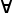# Maths - Types

Type Name Type Constructor Constructor Eliminator
for more details click on link below formation constructors deconstructors notes
0
 0:type

 e:0 A:type absurd(e) : A

empty
cannot be constructed
initial object

1
 1:type
 *:1

unit
cannot be deconstructed
terninal object

Bool   True
False
conditional expression
if then else

inductive datatypes     induction principle
Nat   Z
S n

elim_Nat: (P : Nat -> Set) -> P Z ->
((k : Nat) -> P k -> P (S k)) ->
(n : Nat) -> P n

where:

• P n = property of number n
• Z = zero
• S n = successor to n

elim_Nat P pz ps Z = pz
elim_Nat P pz ps (S k) = ps k (elim_Nat P pz ps k)

List   cons car
cdr

Pair Pair (a,b) fst
snd

Function   λ function application
Expression Atom
Equality (≡) : t->t->Type refl : { A : Typei } -> ( x : A ) -> x ≡ x

J : { A :Typei } ->
(P: (x y :A) -> x ≡ y -> Typej ) ->
(x . P x x ( refl x )) ->{ x y } . ( eq : x ≡ y ) ->P x y eq

+       sum
Record   tuple projection
×       product - special case
of dependant product
Π       dependant product
space of sections
Σ       dependant sum

### Sum, Product and Exponent

A type theory with Sum, Product and Exponent type is related to a Cartesian Closed Category (CCC).

A term may be represented by some variable such as x,y... or a compound term using the following:

If 'M' and 'N' are valid terms, then the following are also valid terms:

Type Term
M[x] We will use this notation to denote a term 'M' with multiple values depending on the value 'x'.
Sum A \/ B <M,N>

This is a union of the two types. An instance will contain an instance of only one of the types.

We can recover the contained types as follows:

• π1(M)
• π2(M)
Product A /\ B (M N) This contains both of the types (like tuples) . An instance will contain an instance of all of the types.
Exponent A->B λ x:M.N

This is a function from M to N.

where x is an instance of 'M' and the function returns an instance of 'N'

See lambda calculus.

Where:

• M,N are terms, that is a base type or a compound type made up as some combination of the above. That is, they are inductively defined.
• x is an instance of 'M'

## Connectives

Conjunction and Disjunction called connectives.

## Relationship to Category Theory

Category theory is deeply related to type theory.

On this page is a discusion about how an object can be defined from a diagram. So can we define a type from a diagram?

Book Shop - Further reading.

Where I can, I have put links to Amazon for books that are relevant to the subject, click on the appropriate country flag to get more details of the book or to buy it from them.Computation and Reasoning - This book is about type theory. Although it is very technical it is aimed at computer scientists, so it has more discussion than a book aimed at pure mathematicians. It is especially useful for the coverage of dependant types.